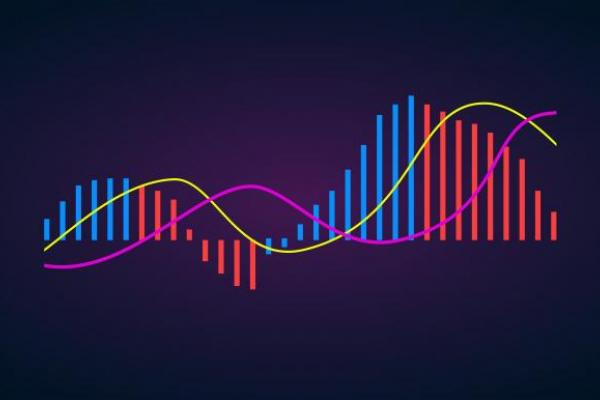# Moving Average: Lots of Signals

Moving average is one of the most effective and used indicators. We can say it’s a basic of technical analysis, as it serves not only as a unique indicator but as a part of other technical tools. Every trader should know how to apply moving averages to the chart. In our guide, we will tell you all the secrets of this mathematical instrument.

## Moving Average: How to Determine

The moving average is a trend indicator that is represented by one line. It's located on the price chart, not in the separate window, like many other indicators. The primary function is to determine the direction of the trend. At the same time, the tool can be used to place support and resistance levels. Although the moving average is a one-line indicator, to get signals, traders use at least two lines with different periods.

## Moving Averages: Types

There are four types of this indicator. They differ depending on what they are used for.

### Simple (SMA)

It's the most used type of moving average indicators. Simple moving average reflects the average of the close prices regarding the period you choose. It means that the prices used for the calculation are equal in their meaning for the indicator’s calculation.

For instance, we implement a 200-day MA. To calculate the Simple MA, we need to add the values of 200 prices and divide the result by 200.

### Exponential (EMA) and Linear Weighted

Although it's two separate types, we combined them as they are almost identical. These types put weight on the latest prices, adding a higher coefficient in the calculation.

Such a particular calculation helps the MA to resemble the price movements more accurately. Moreover, the signals appear faster. Here you should be picky and choose the right signs. Later, we will explain how to combine the MA indicator with other technical tools to get useful alerts.

### Smoothed

Smoothed MA is the most effective when defining a trend as it smooths the fluctuation of the price the most. This type is based on the Simple moving average.

## Moving Average Indicator in MetaTrader

As the moving average is one of the leading technical tools, it's a standard indicator implemented in MetaTrader. To apply it to your chart, you need to click "Insert", then "Indicators". After that, find "Trend" indicators, and choose the necessary instrument.

After you click on the indicator, a setting window will appear. Here you can choose the type of moving average, period, price, and shift.

### Period

A period refers to the number of candles that will be used to calculate the moving average. The period determines the number of signals you will get. A more significant period will smooth the market movements, so there will be fewer signals. It can prevent you from the wrong entry and exit points. At the same time, if you trade on a small timeframe, there are risks you will miss a good alert.

Contrary, a shorter period increases the number of signals. However, there are risks of fake signals. So, confirmation is necessary.

Usually, investors use 50, 100, 200 periods when trading on high timeframes and 9, 12, 26 periods on low timeframes.

1. 200-period MA
2. 50-period MA
3. 20-period MA
4. 10-period MA

Use a more considerable period of moving average on higher timeframes (from H4). Set a smaller period of moving average on lower timeframes (up to H1).

### Price

As for prices, you can choose from open, close, low, high, median, typical, and weighed close. Still, most of the traders apply the close price setting.

### Shift

Shift allows traders to move the moving average back or forth. This setting can be applied to a specific trading strategy. However, investors usually don't change the shift.